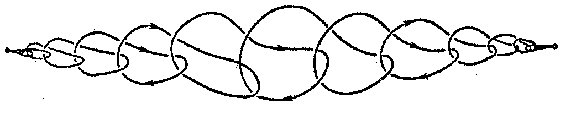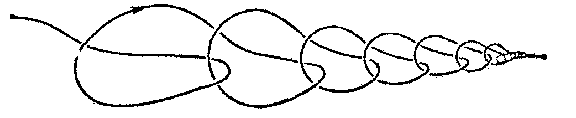# Wild sphere

A closed manifold in Euclidean three-space \$E^3\$ obtained by a wild imbedding of the sphere \$S^2\$ in \$E^3\$. Thus, a wild sphere is the sum of two discs with a common boundary, which is a wild knot. The first example of a wild sphere is the so-called "horned sphere of Alexander", horned sphere or Alexander sphere (Fig. a); it bounds a domain which is not homeomorphic to \$E^3\$ (in the figure this is the interior of the cylinder without any interlinking handles and points forming their boundary). Fig. b shows a wild sphere in which the exterior domain alone is not homeomorphic to \$E^3\$.Figure: w097990aFigure: w097990b

How to Cite This Entry:
Wild sphere. Encyclopedia of Mathematics. URL: http://encyclopediaofmath.org/index.php?title=Wild_sphere&oldid=53888
This article was adapted from an original article by M.I. Voitsekhovskii (originator), which appeared in Encyclopedia of Mathematics - ISBN 1402006098. See original article